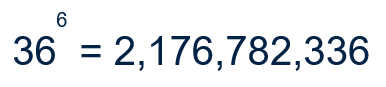# SILO 6.4 (DRAFT)

## Year 6, Term 4: Nanotechnology

### Learning intention: Students convert between metric units of length, mass and capacity and make relevant connections to the properties of water.

Overview: Nanotechnology involves the manipulation of matter on a near-atomic scale which might suggest that this is beyond what Year 6 students can investigate, but certain principles such as the ratio between surface area and volume are manageable and relevant for this age group.  These concepts are scaffolded by looking at the metric system, calibration and various scales used in science, namely; ordinal, linear and logarithmic.
NSW Syllabus
Australian Curriculum (version 9.0)
"A student selects and uses the appropriate unit to estimate, measure and calculate volumes and capacities." (MA3-3DS-02)
"Students learn to convert between common metric units of length, mass and capacity; choose and use decimal representations of metric measurements relevant to the context of a problem." (AC9M6M01)

## Week 1

Introduction to the topic

A nanometre is one billionth of a metre (i.e., one millionth of a millimetre).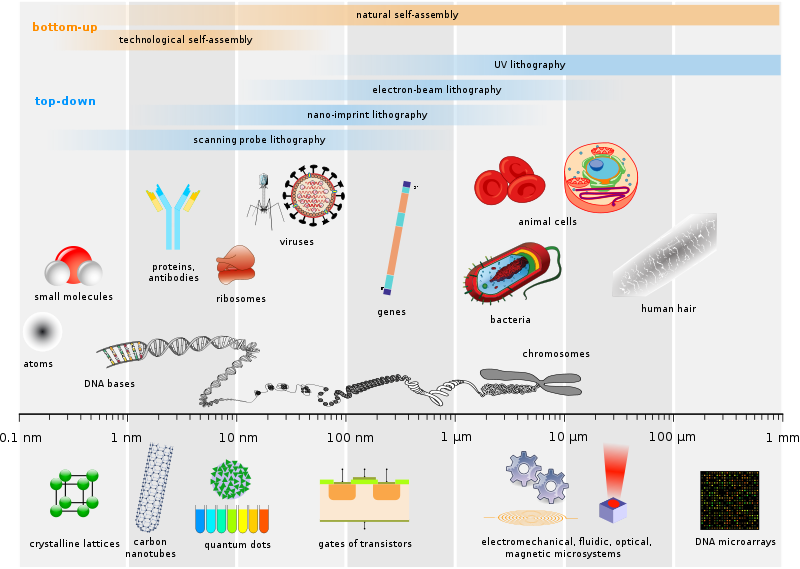(CC BY-SA 2.5 Source: https://commons.wikimedia.org/wiki/File:Biological_and_technological_scales_compared-en.svg)

This video (3:29) explains some of the ways in which nanotechnology is at the cutting edge of science and technology.

## Week 2

The logic behind the metric system

The properties of water

Water freezes at 0 degrees Celsius and boils at 100 degrees.  This means that the calibration of the Celsius scale is based on water.• The temperature inside a refrigerator is generally set at 4 degrees Celsius.
• The human bodies are generally around 37 degrees Celsius.

Water was used as the basis for quantifying mass according to volume.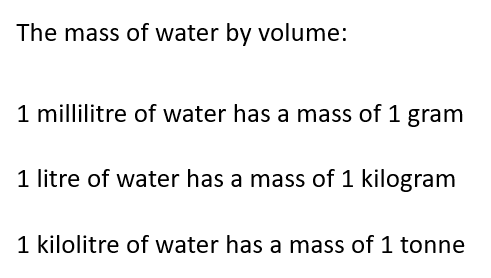## Week 3

Introduction to exponents

### Q: How many combinations are there with six-character numbers plates?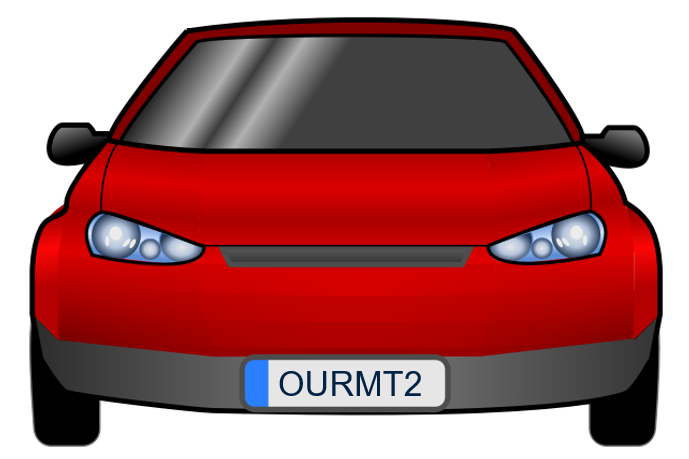## Week 4

Exploring the ratio of surface area to volume

The main thing to note here is that the ratio of surface area to volume is not fixed as shown in the figure below.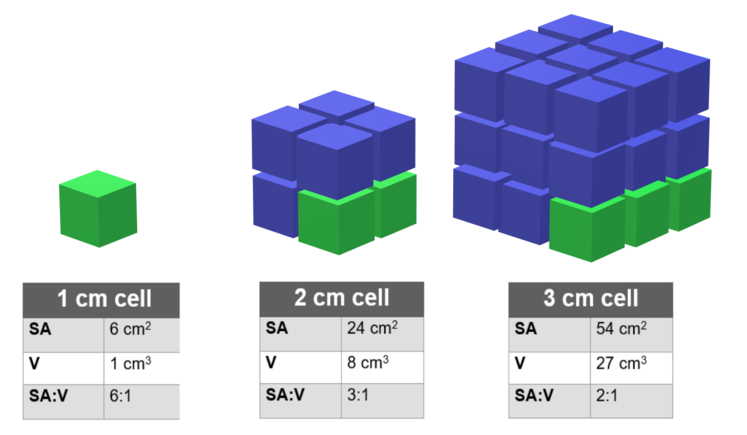There are many examples of this principle in nature.  For example:

• Chewing food into smaller pieces aids digestion as the surface area is increased while the volume remains the same.
• Your fingers are the first parts of your body to get cold as they have a high ratio of surface area to volume.
• Elephants have large ears to help them keep cool as their ears also have a high ratio of surface area to volume.

The ratio of surface area to volume can also be seen in everyday phenomena.  For example, when building a fire it is common to use small pieces of wood first which is know as kindling.  Because the kindling is small, it has a larger surface ares compared to its volume.

The following video (2:26) from the Siteman Cancer Center contains two examples of how nanotechnology is being used in the field of medical research to fight cancer.

## Week 5

Introduction to scales

Ordinal scales

An ordinal scale has a non specified degree of variation.  In other words, the scale is somewhat arbitrary.  An example is the Mohs scale of mineral hardness introduced in 1822 by the German geologist and mineralogist Friedrich Mohs.

### Q: Is there a direct correlation?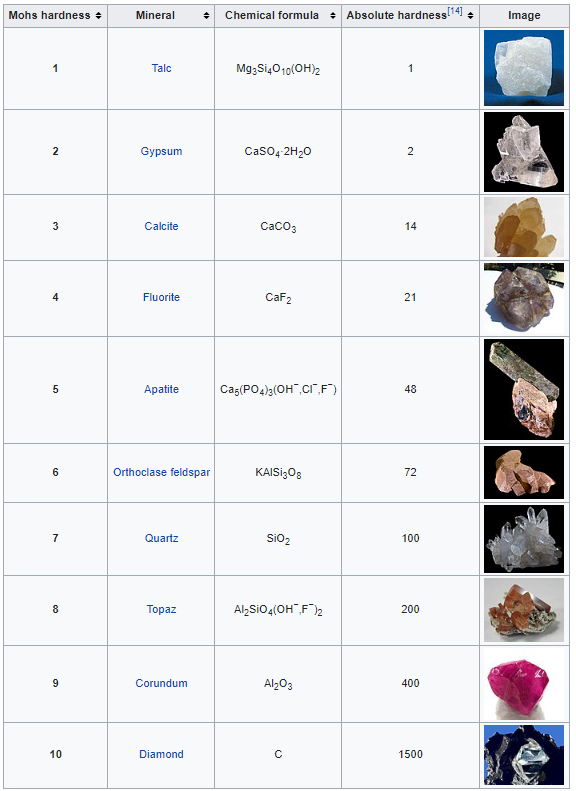https://en.wikipedia.org/wiki/Mohs_scale_of_mineral_hardness

## Week 6

Linear scales

A linear scale has a specified degree of variation.  In other words, there is a direct mathematical correlation along the scale due to the way that it has been calibrated.  An example is the Celsius scale.

## Week 7

Logarithmic scales

In a logarithmic scale, each interval is increased by a factor of the base of the logarithm, which is often a multiple of 10. Examples of common logarithmic scales are pH (to measure acidity), decibels (sound intensity), and the Richter scale (earthquakes).https://commons.wikimedia.org/wiki/File:2713_pH_Scale-01.jpg

Revision of scales

## Week 8

Calibration

Calibration is the process of configuring an instrument to improve the accuracy of measurements and readings.

The following video (1:39) uses calibration to improve the accuracy of a digital compass.

## Week 9

The International system of units

## Week 10

Moderated self-assessment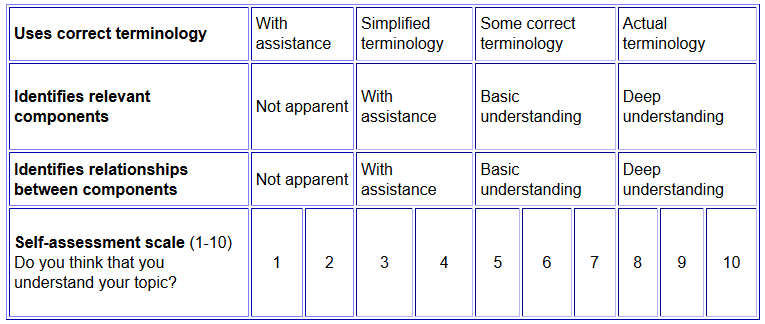------------------

Answer to the exponents question about combinations with six character number plates: